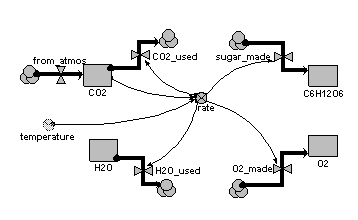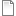# Modelling chemical reactions: CO2 + H2O → Sugar and O2

ModelId:
chemical1
SimileVersion:
5.x

On the face of it, System Dynamics should be a good notation for modelling chemical reactions: chemical reactions are continuous processes involving amounts of substances, and System Dynamics is suitable for modelling continuous processes involvinga mounts of substances.

However, the box-and-arrow notation of System Dynamics has a different meaning than a box-and-arrow notation for chemical reactions. In the former, two compartments connected by a flow should be expressed in the same units (e.g. kilograms of carbon). In the latter, the diagram shows the combination/conversion of substances.

One way of handling this is to have a separate compartment for each substance (element or compound), with each compartment having a flow in from the source/sink and a flow out to the source/sink. The flows involved in a particular reaction are then all controlled by a single rate variable, with each flow being expresesed in terms of the appropriate number of atoms or molecules that participate in the reaction.Equations:

Compartments:

CO2: initial value = 100
H2O: initial value = 100
C6H12O6: initial value = 0
O2: initial value = 0

Flows:
CO2_used = 6*rate
H2O_used = 6*rate
from_atmos = 0

Variables:
rate = 0.01*CO2*2*(temperature/10)

Results:AttachmentSizechemical1.sml174.99 KB
Model tags: